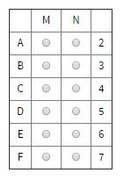• NEW! FREE Beat The GMAT QuizzesHundreds of Questions Highly Detailed Reporting Expert Explanations
• 7 CATs FREE!
If you earn 100 Forum Points

Engage in the Beat The GMAT forums to earn
100 points for $49 worth of Veritas practice GMATs FREEVERITAS PRACTICE GMAT EXAMS Earn 10 Points Per Post Earn 10 Points Per Thanks Earn 10 Points Per Upvote A fair coin has 2 distinct flat sides-one of which bears t This topic has 1 expert reply and 0 member replies Top Member A fair coin has 2 distinct flat sides-one of which bears tA fair coin has 2 distinct flat sides-one of which bears the image of a face and the other of which does not-and when the coin is tossed, the probability that the coin will land faceup is $$\frac{1}{2}$$. For certain values of M, N, p, and q, when M fair coins are tossed simultaneously, the probability is p that all M coins land faceup, and when N fair coins are tossed simultaneously, the probability is q that all N coins land face up. Furthermore, $$M < N$$ and $$\frac{1}{p} + \frac{1}{q} = 72$$. In the table, select a value for $$M$$ and a value for $$N$$ that are jointly consistent with the given information. Make only two selections, one in each column.The OA is M=3 N=6 Source: Official Guide GMAT/MBA Expert GMAT InstructorJoined 04 Dec 2012 Posted: 2094 messages Followed by: 238 members Upvotes: 1443 If one coin flip has a 1/2 chance of landing face up, then the probability that two coin flips will both be face up is $$\frac{1}{2}\cdot\frac{1}{2}=\frac{1}{4}$$ Thus, for any M coin flips, the probability of all flips landing face up is $$p=\frac{1}{2^M}$$ , and for any N coin flips, the probability of all flips landing face up is $$q=\frac{1}{2^N}$$ . We're told that $$\frac{1}{p} + \frac{1}{q} = 72$$ , so we can infer that $${2^M}+{2^N}=72$$ . Think of powers of 2 that would add to 72, starting with the largest power of 2 less than 72: $${2^6}$$ --> 64 + 8 = 72 --> yes! These are both powers of 2. $${2^5}$$ --> 32 + 40 = 72 --> no, 40 is not a power of 2. $${2^4}$$ --> 16 + 56 = 72 --> no, 56 is not a power of 2. $${2^3}$$ --> 8 + 64 = 72 --> yes, we already know this works. $${2^2}$$ --> 4 + 68 = 72 --> no, 68 is not a power of 2. $${2^1}$$ --> 2 + 70 = 72 --> no, 70 is not a power of 2. The only two numbers that work are $${2^6}$$ and $${2^3}$$ , 64 and 8. Since we're given the constraint that M must be less than N, $${2^M}$$ must equal $${2^3}$$ and $${2^N}$$ = $${2^6}$$ . Thus, M=3 and N=6 . _________________Ceilidh Erickson Manhattan Prep GMAT & GRE instructor EdM in Mind, Brain, and Education Harvard Graduate School of Education Manhattan Prep instructors all have 99th+ percentile scores and expert teaching experience. Sign up for a FREE TRIAL, and learn why we have the highest ratings in the GMAT industry! Free Manhattan Prep online events - The first class of every online Manhattan Prep course is free. Classes start every week. •Get 300+ Practice Questions 25 Video lessons and 6 Webinars for FREE Available with Beat the GMAT members only code •5-Day Free Trial 5-day free, full-access trial TTP Quant Available with Beat the GMAT members only code •Free Veritas GMAT Class Experience Lesson 1 Live Free Available with Beat the GMAT members only code •Award-winning private GMAT tutoring Register now and save up to$200

Available with Beat the GMAT members only code

•5 Day FREE Trial
Study Smarter, Not Harder

Available with Beat the GMAT members only code

•Magoosh
Study with Magoosh GMAT prep

Available with Beat the GMAT members only code

•FREE GMAT Exam
Know how you'd score today for \$0

Available with Beat the GMAT members only code

•Free Trial & Practice Exam
BEAT THE GMAT EXCLUSIVE

Available with Beat the GMAT members only code

•1 Hour Free
BEAT THE GMAT EXCLUSIVE

Available with Beat the GMAT members only code

•Free Practice Test & Review
How would you score if you took the GMAT

Available with Beat the GMAT members only code

Top First Responders*

1Ian Stewart 53 first replies
2Brent@GMATPrepNow 36 first replies
3Jay@ManhattanReview 29 first replies
4GMATGuruNY 25 first replies
5ceilidh.erickson 15 first replies
* Only counts replies to topics started in last 30 days
See More Top Beat The GMAT Members

Most Active Experts

1Scott@TargetTestPrep

Target Test Prep

199 posts
2Max@Math Revolution

Math Revolution

90 posts
3Brent@GMATPrepNow

GMAT Prep Now Teacher

76 posts
4Ian Stewart

GMATiX Teacher

62 posts
5GMATGuruNY

The Princeton Review Teacher

44 posts
See More Top Beat The GMAT Experts